#### 期刊菜单

Study on Phosphorus Fixation Properties of Modified Iron Bearing Clay Ore
DOI: 10.12677/AEP.2023.131012, PDF, HTML, XML, 下载: 30  浏览: 82

1. 引言

2. 实验部分

2.1. 试剂和材料Table 1. Composition of iron bearing clay ore

2.2. 分析方法

2.3. 溶液初始pH影响实验

2.4. 共存离子影响实验

2.5. 等温吸附实验与动力学吸附实验

1) 等温吸附实验

0.2 g 500-CO与100 mL不同浓度的磷酸盐溶液(P 10~40 mg/L)充分混合后在恒温下水浴振荡(200 rpm/min，25℃)2 h后测定溶液中剩余磷酸盐的浓度(均以P计)。0.14 g M-CO与100 mL不同浓度的磷酸盐溶液(P 10~50 mg/L)充分混合后在恒定的温度下水浴振荡(200 rpm/min，25℃) 1 h后测定溶液中剩余磷浓度。

${q}_{e}=\frac{\left({C}_{0}-{C}_{e}\right)V}{m}$ (1)

Langmuir和Freundlich等温吸附模型：

${q}_{e,L}=\frac{{K}_{L}{q}_{L,m}{C}_{e}}{1+{K}_{L}{C}_{e}}$ (2)

${q}_{e,F}={K}_{F}{C}_{e}^{n}$ (3)

2) 动力学吸附实验

0.2 g 500-CO与100 mL磷酸盐溶液(P 10 mg/L)充分混合后在恒温下水浴振荡(200 rpm/min，25℃)，分别于5，10，20，30，60，120 min的时间点取样测定磷酸盐的浓度(均以P计)。0.14 g M-CO与100 mL磷酸盐溶液(P 10 mg/L)充分混合后在恒温下水浴振荡(200 rpm/min，25℃)，分别于2，5，10，20，30，60 min的时间点取样测定磷酸盐的浓度。

$\mathrm{ln}\left({q}_{e}-{q}_{t}\right)=\mathrm{ln}{q}_{e}-{k}_{1}t$ (4)

$\frac{t}{{q}_{t}}=\frac{1}{{k}_{2}{q}_{e}^{2}}-\frac{t}{{q}_{e}}$ (5)

2.6. M-CO的磷酸根脱附实验

3. 结果与讨论

3.1. 投加量实验

3.2. 溶液初始pH对磷酸盐吸附影响实验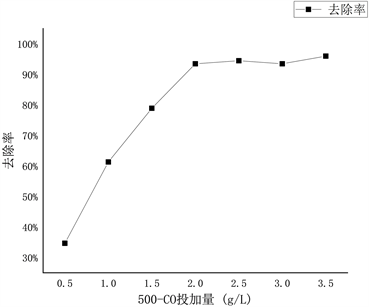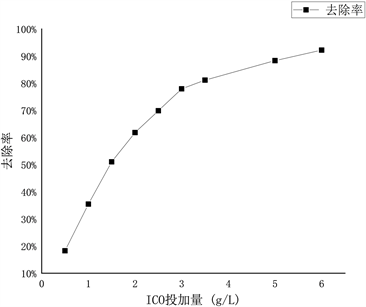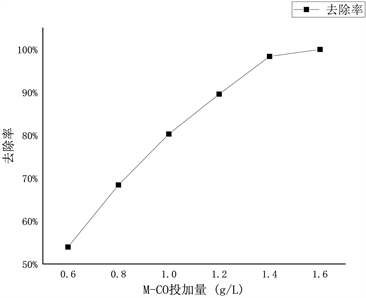Figure 1. Material dosage curve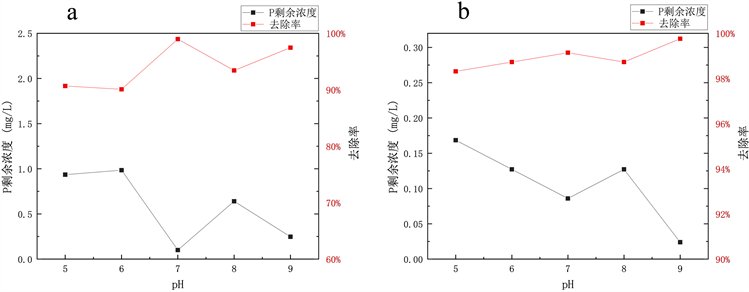Figure 2. Effect of initial pH of solution on phosphate adsorption (a) 500-CO; (b) M-CO

${\text{2Fe}}^{\text{3}+}+\left(\text{3}+x\right){\text{H}}_{\text{2}}\text{O}\to {\text{Fe}}_{\text{2}}{\text{O}}_{\text{3}}\cdot x{\text{H}}_{\text{2}}\text{O}+{\text{6H}}^{+}$ (6)

${\text{Fe}}^{\text{3}+}+{\text{3H}}_{\text{2}}\text{O}\to \text{Fe}{\left(\text{OH}\right)}_{\text{3}}+{\text{3H}}^{+}$ (7)

${\text{2Fe}}_{\text{2}}{\text{O}}_{\text{3}}\cdot {\text{3H}}_{\text{2}}\text{O}+{\text{3H}}_{\text{2}}{\text{PO}}_{4}^{-}+{\text{3H}}^{+}\to {\text{Fe}}_{\text{4}}{\left(\text{OH}\right)}_{\text{3}}{\left({\text{PO}}_{\text{4}}\right)}_{\text{3}}+{\text{9H}}_{\text{2}}\text{O}$ (8)

$\text{4Fe}{\left(\text{OH}\right)}_{\text{3}}+{\text{H}}_{\text{2}}{\text{PO}}_{4}^{-}\to {\text{Fe}}_{\text{4}}{\left(\text{OH}\right)}_{\text{3}}{\left({\text{PO}}_{\text{4}}\right)}_{\text{3}}$ (9)

${\text{2Fe}}^{\text{3}+}+{\text{3H}}_{\text{2}}{\text{PO}}_{4}^{-}\to {\text{Fe}}_{\text{2}}{\left({\text{HPO}}_{\text{4}}\right)}_{\text{3}}+{\text{3H}}^{+}$ (10)

${\text{Fe}}_{\text{2}}{\left({\text{HPO}}_{\text{4}}\right)}_{\text{3}}+{\text{Fe}}^{\text{3}+}\to {\text{3FePO}}_{\text{4}}+{\text{3H}}^{+}$ (11)

${\text{2Fe}}^{\text{3}+}+{\text{6OH}}^{-}\to {\text{Fe}}_{\text{2}}{\text{O}}_{\text{3}}\cdot {\text{3H}}_{\text{2}}\text{O}$ (12)

${\text{2Fe}}^{\text{3}+}+{\text{6OH}}^{-}\to \text{Fe}{\left(\text{OH}\right)}_{\text{3}}$ (13)

${\text{2Fe}}_{\text{2}}{\text{O}}_{\text{3}}\cdot {\text{3H}}_{\text{2}}\text{O}+{\text{3H}}_{\text{2}}{\text{PO}}_{4}^{-}+{\text{3H}}^{+}\to {\text{Fe}}_{\text{4}}{\left(\text{OH}\right)}_{\text{3}}{\left({\text{PO}}_{\text{4}}\right)}_{\text{3}}+{\text{9H}}_{\text{2}}\text{O}$ (14)

$\text{4Fe}{\left(\text{OH}\right)}_{\text{3}}+{\text{H}}_{\text{2}}{\text{PO}}_{4}^{-}\to {\text{Fe}}_{\text{4}}{\left(\text{OH}\right)}_{\text{3}}{\left({\text{PO}}_{\text{4}}\right)}_{\text{3}}$ (15)

${\text{2Fe}}^{\text{3}+}+{\text{2H}}_{\text{2}}{\text{PO}}_{4}^{-}+{\text{4OH}}^{-}\to {\text{FePO}}_{\text{4}}+{\text{4H}}_{\text{2}}\text{O}$ (16)

3.3. 共存离子影响实验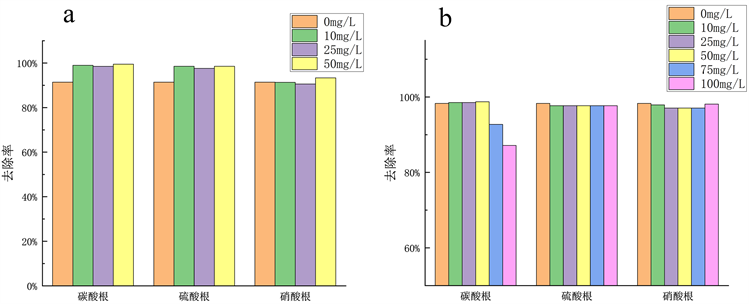Figure 3. Effect of coexisting ions on phosphate adsorption by (a) 500-CO and (b) M-CO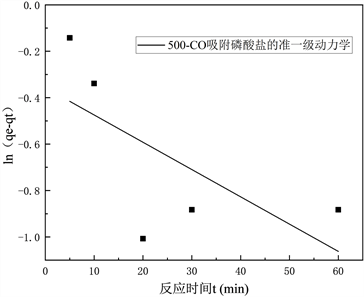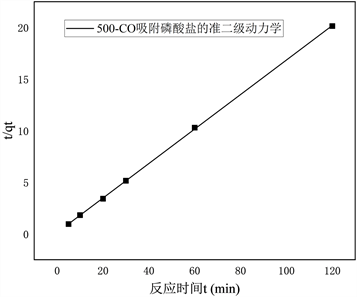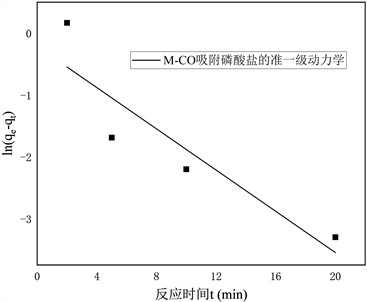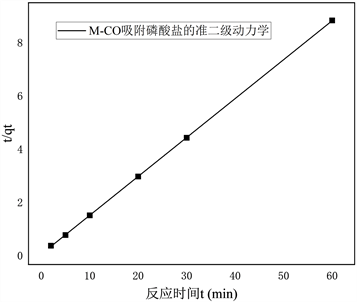Figure 4. Adsorption kinetics of 500-CO and M-CO

3.4. 吸附动力学与等温吸附模型500-CO和M-CO的Langmuir等温吸附模型与Freundlich等温吸附模型拟合结果如图5所示。根据表2中的拟合参数，500-CO和M-CO的Langmuir等温吸附模型拟合的R2分别为0.97144和0.9686，大于Freundlich等温吸附模型的R2 = 0.90993和0.91323，这表明500-CO和M-CO吸附磷酸盐都更倾向于单分子层吸附。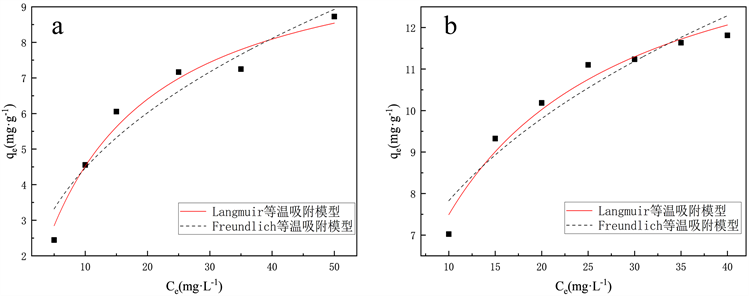Figure 5. Isothermal adsorption model (a) 500-CO; (b) M-CO

3.5. M-CO的磷酸根脱附实验

M-CO的脱附实验如图6所示，在100 mg/L ${\text{C}}_{\text{2}}{\text{O}}_{4}^{2-}$ 溶液中，材料吸附的P有60%以上被脱附出来。在pH = 9的水溶液中，吸附磷酸盐M-CO (原吸附量 = 6.94 mg/g)脱附量很少，而在pH = 11的水溶液中，60 min时的脱附量达到4.38 mg/g，脱附率达63%。磷酸盐主要与材料表面的Fe结合生成难溶配体，被吸附到吸附剂表面， ${\text{C}}_{\text{2}}{\text{O}}_{4}^{2-}$ 和材料表面的Fe结合生成草酸铁，能与磷酸盐产生竞争吸附，将磷酸盐脱附出来。在pH = 9时，溶液中的OH-会先与材料中未反应完的铁结合，因此脱附量低。在pH = 11时，强碱性条件下过量的OH在溶液中能与磷酸盐形成竞争吸附，将磷酸盐和铁的配合物转化为Fe(OH)3，从而将材料表面吸附的磷酸盐释放出来。这说明M-CO吸附的磷酸盐在一定条件下可以再次释放出来，这为吸附磷酸盐后M-CO材料的利用提供了方向，比如施入土壤中作为磷肥。

3.6. SEM表征

3.7. XRD与XPS表征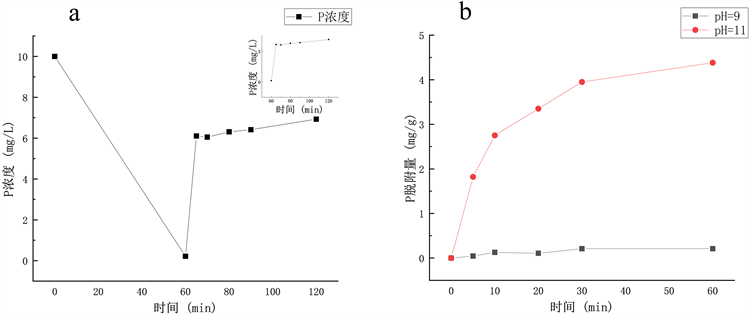Figure 6. Desorption curves of M-CO in (a) 100 mg/L ${\text{C}}_{\text{2}}{\text{O}}_{4}^{2-}$ and (b) alkaline aqueous solutions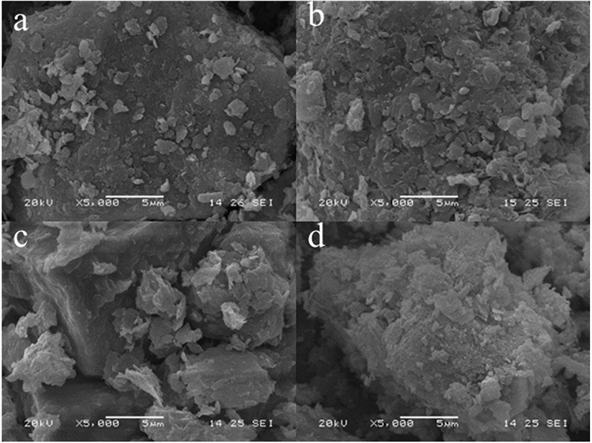Figure 7. SEM diagram (a) ICO; (b) 500-CO; (c) M-CO; (d) M-CO after phosphate adsorption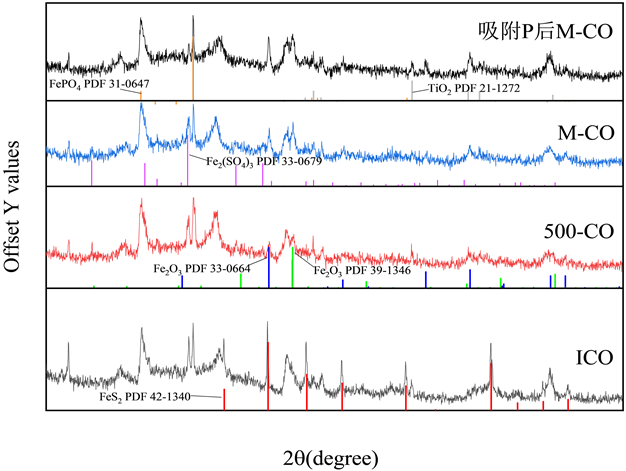Figure 8. XRD patterns of ICO, 500-CO and M-CO before and after adsorption of P

M-CO的P 2p XPS谱图中未能观察到关于P的谱峰，吸附磷酸盐后，M-CO的P 2p XPS谱图中结合能约133.9 eV处出现P-2p谱峰，说明P成功吸附在材料表面。经拟合，结合能位于133.53 eV、134.3 eV的P-2p峰分别与 ${\text{PO}}_{4}^{3-}$${\text{HPO}}_{4}^{2-}$ 对应   。这说明在反应过后材料表面吸附了磷酸根基团，这与XRD的分析一致。Figure 9. P 2p XPS spectrum (a) M-CO; (b) M-CO after phosphate adsorption

ICO的Fe 2p XPS谱图中存在一个结合能位于707 eV附近的Fe 2p谱峰，与FeS2中的Fe(Ⅱ)结合能相匹配，而在500-CO和M-CO的谱图中未能观察到此峰，说明两种改性材料均将FeS2氧化。Fe 2p XPS谱图中位于712 eV和725 eV附近的峰分别对应Fe(Ⅲ)的Fe 2p3/2峰和Fe 2p1/2峰，此外，ICO中位于710 eV附近和500-CO、M-CO中位于715 eV附近的Fe 2p峰，都对应Fe(Ⅱ)的Fe 2p3/2峰      ，在改性后，Fe(Ⅱ)的结合能发生改变，这可能是材料改性过程不仅使材料中的部分Fe(Ⅱ)氧化为 Fe(Ⅲ)，还使原有的部分Fe(Ⅱ)结合形式发生改变。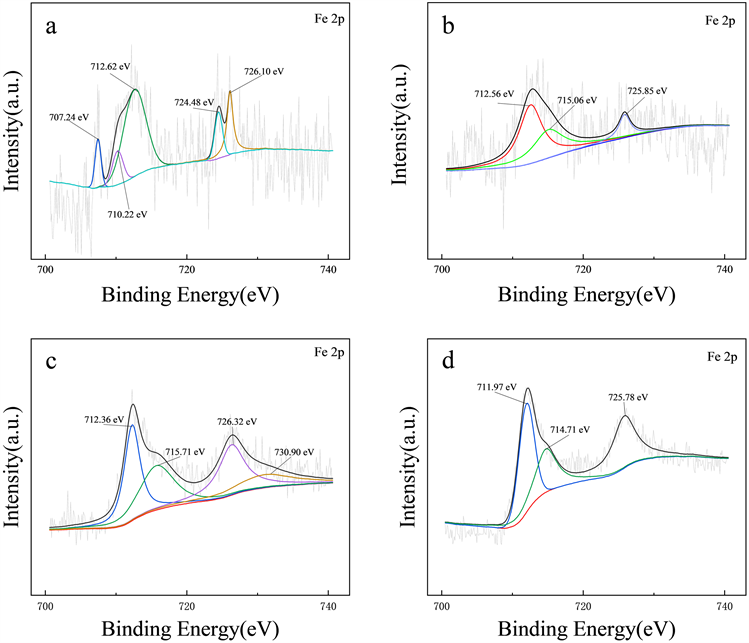Figure 10. Fe 2p XPS spectrum (a) ICO; (b) 500-CO; (c) M-CO; (d) M-CO after phosphate adsorption

4. 结论

1) 通过将含铁粘土原矿ICO进行热改性和碱/热复合改性，制备出500-CO和M-CO，对低浓度磷酸盐溶液(P 10 mg/L)进行吸附固定，均实现了90%以上的P去除率，500-CO和M-CO最大理论吸附量(以P计)分别为10.98 mg/g，15.14 mg/g。

2) ICO材料改性后，表面的Fe(Ⅲ)对磷酸盐的吸附起主要作用，对磷酸盐的吸附类型为单分子层化学吸附，吸附量随初始pH在5~9内增加有少量提升。

3) 共存离子 ${\text{SO}}_{4}^{2-}$${\text{NO}}_{3}^{-}$${\text{CO}}_{3}^{2-}$ 对磷酸盐吸附量影响较小。 ${\text{C}}_{\text{2}}{\text{O}}_{4}^{2-}$ 和强碱性条件均能使M-CO实现脱附，表明M-CO在低浓度磷废水中，能实现磷回收。

4) 实现了对粘土原矿的利用，降低成本，对水体磷污染控制具有实践价值。

NOTES

*第一作者。

#通讯作者。

  陈伟江, 廖月清, 王苗苗, 等. 水体富营养化修复技术研究进展[J]. 应用化工, 2022, 51(2): 531-537.  申璐, 刘伟玲, 邓陈宁, 等. 长江流域总磷污染治理相关政策、问题与对策[J]. 环境保护, 2022, 50(17): 37-40.  嵇晓燕, 彭丹. “十三五”时期长江流域总磷浓度变化特征[J]. 长江科学院院报, 2022, 39(8): 1-9.  尹炜, 王超, 张洪. 长江流域总磷问题思考[J]. 人民长江, 2022, 53(4): 44-52.  井柳新, 马乐宽, 孙宏亮, 等. “十四五”时期长江流域总磷管控重点及施策方向解析[J]. 环境保护, 2022, 50(17): 48-51.  Xie, F.Z., Wu, F.C., Liu, G.J., et al. (2014) Removal of Phosphate from Eutrophic Lakes through Adsorption by in Situ Formation of Magnesium Hydroxide from Diatomite. Environmental Science & Technology, 48, 582-590. https://doi.org/10.1021/es4037379  李迎春, 董良飞, 仝驰, 等. 稀土改性凹凸棒土对低浓度磷的吸附性能[J]. 环境工程学报, 2021, 15(10): 3214-3222.  Zamparas, M., Deligiannakis, Y. and Zacharias, I. (2013) Phosphate Adsorption from Natural Waters and Evaluation of Sediment Capping Using Modified Clays. Desalination and Water Treatment, 51, 2895-2902. https://doi.org/10.1080/19443994.2012.748139  汪逸云, 尹洪斌, 孔明, 等. 镧铝改性凹凸棒粘土对富营养化湖泊有机磷控制效果[J]. 中国环境科学, 2020, 40(9): 3801-3809.  Akin, I.D. and Likos, W.J. (2020) Rela-tionship between Water Vapor Sorption Kinetics and Clay Surface Properties. Journal of Geotechnical and Geoenvironmental Engineering, 146, 6. https://doi.org/10.1061/(ASCE)GT.1943-5606.0002337  Delavi, D.G.G., De Noni, A. and Hotza, D. (2013) Deflocculant Consumption of Clay Suspensions as a Function of Specific Surface Area and Cation Exchange Capacity. Clay Minerals, 48, 473-480. https://doi.org/10.1180/claymin.2013.048.3.04  Bacelo, H., Pintor, A.M.A., Santos, S.C.R., et al. (2020) Per-formance and Prospects of Different Adsorbents for Phosphorus Uptake and Recovery from Water. Chemical Engi-neering Journal, 381, 18. https://doi.org/10.1016/j.cej.2019.122566  张英, 覃武林, 孙伟, 等. 石灰和氢氧化钠对黄铁矿浮选抑制的电化学行为[J]. 中国有色金属学报, 2011, 21(3): 675-679.  徐垚, 李大鹏, 韩菲尔. CaO2同步氧化Fe(Ⅱ)除磷的研究[J]. 工业水处理, 2018, 38(3): 21-24.  Smith, S., Takacs, I., Murthy, S., et al. (2008) Phosphate Complexation Model and Its Implications for Chemical Phosphorus Removal. Water Environment Research, 80, 428-438. https://doi.org/10.1002/j.1554-7531.2008.tb00349.x  Qiao, L.P., Lou, J., Zhang, S.F., et al. (2016) The Entrance Mechanism of Calcium and Phosphorus Elements into Micro Arc Oxidation Coatings Developed on Ti6Al4V Alloy. Surface & Coatings Technology, 285, 187-196. https://doi.org/10.1016/j.surfcoat.2015.11.041  Wang, W., Mohammadi, F. and Alfantazi, A. (2012) Corrosion Behaviour of Niobium in Phosphate Buffered Saline Solutions with Different Concentrations of Bovine Serum Albumin. Corrosion Science, 57, 11-21. https://doi.org/10.1016/j.corsci.2011.12.039  Hu, W., Li, M.X., Chen, T.H., et al. (2018) Enrichment of U(Ⅵ) on Bacillus subtilis/Fe3O4 Nanocomposite. Journal of Molecular Liquids, 258, 244-252. https://doi.org/10.1016/j.molliq.2018.03.021  Yamashita, T. and Hayes, P. (2008) Analysis of XPS Spectra of Fe2+ and Fe3+ Ions in Oxide Materials. Applied Surface Science, 254, 2441-2449. https://doi.org/10.1016/j.apsusc.2007.09.063  Cui, Q.L., Xu, J.L., Wang, W., et al. (2020) Phosphorus Re-covery by Core-Shell γ-Al2O3/Fe3O4 Biochar Composite from Aqueous Phosphate Solutions. Science of the Total En-vironment, 729, 10. https://doi.org/10.1016/j.scitotenv.2020.138892  Fanlo, I., Gervilla, F., Mateo, E., et al. (2008) X-Ray Photo-electron Spectroscopy Characterization of Natural Chromite from Mercedita Mine (Eastern Cuba): Quantification of the Fe3+/Fe2+ Ratio. European Journal of Mineralogy, 20, 125-129. https://doi.org/10.1127/0935-1221/2008/0020-1794  Ghods, P., Isgor, O.B., Brown, J.R., et al. (2011) XPS Depth Profiling Study on the Passive Oxide Film of Carbon Steel in Saturated Calcium Hydroxide Solution and the Effect of Chloride on the Film Properties. Applied Surface Science, 257, 4669-4677. https://doi.org/10.1016/j.apsusc.2010.12.120